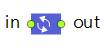AnyLogic

# FluidConvertThe output rate of this block always equals the input rate multiplied by a given "conversion factor". The factor can be both greater than 1, or less than 1, or 1, so FluidConvert can both amplify or diminish the flow. Note, that if the rate value falls below the value of Utils.RATE_TOLERANCE constant, i.e. 1.0e-9, it will be snapped to 0.

The block can be used to model, for example, packaging or unpackaging fluid or items. Typically, this assumes change of flow units.

FluidConvert is a zero-capacity block, it does not contain any amount of fluid.

The output batch can be the same as the input batch, or a custom batch (which may depend on the input batch).

As any other block the FluidConvert block allows you to react to new batches appearing at the input.

## Parameters

Factor
Multiplier that is applied to the input rate. Must be a positive number.
Syntax: double factor
Default value: 1
Set new value at runtime: set_factor(new value)
Custom output batch
If the option is selected (the value is true), the output batch will be defined by the Output batch parameter below. Otherwise, it will be the same as the input batch.
Syntax: boolean modeBatchCustom
Set new value at runtime: set_modeBatchCustom(new value)
###### Output batch
[Visible and applies only if the Custom output batch option is set] Defines the output batch, which may depend on the input batch.
Type of the value: Object
Local variable: Object batchIn — the input batch
Custom batch color
[Visible and applies only if the Custom output batch option is set] If set, the output batch color is defined by the Batch color parameter below, otherwise the standard color mapping applies.
Syntax: boolean customBatchColor
Set new value at runtime: set_customBatchColor(new value)
###### Batch color
[Visible and applies only if the Custom batch color option is set] Defines the color of the output batch.
Type of the value: Color
Local variable: Object batch — the output batch

### Animation

Show batches in flowchart
If set, the block icon will display the current input batch color.
Syntax: boolean showBatchesInFlowchart

### Actions

On new batch
Action executed when a new batch appears at the block input. It will not necessarily start flowing into the block. You can, for example, change the factor in this action.
Local variable: Object batch — the batch
On rate change
Action executed when any of the flow rates (input or output) changes.
Changing something in the action may result in another immediate rate change and immediately following another call of On rate change, so the user can possibly create a livelock.
Local variables:
double inrate — the current input rate
double outrate - the current output rate

## Functions

Fluid amount
Function Description
double amountPassedIn() Returns the total amount of fluid passed through the input port of the pipeline since the start of the simulation.
double amountPassedIn(AmountUnits units) Returns the total amount of fluid (in given units) passed through the input port of the block since the start of the simulation.
double amountPassedOut() Returns the total amount of fluid passed through the output port of the pipeline since the start of the simulation.
double amountPassedOut(AmountUnits units) Returns the total amount of fluid (in given units) passed through the output port of the block since the start of the simulation.
Flow rate
Function Description
double currentRateIn() Returns the current flow rate of fluid that goes in.
double currentRateIn(FlowRateUnits units) Returns the current flow rate (in given units) of fluid at the block input.
double currentRateOut() Returns the current flow rate of fluid that goes out.
double currentRateOut(FlowRateUnits units) Returns the current flow rate (in given units) of fluid at the block output.
Output batch
Function Description
double updateOutputBatch() Forces immediate recalculation of the output batch and its color according to the current parameters settings.
void setBatchOut(Object batch, Color color) Changes the output batch and color while the input batch stays the same. When the new input batch arrives, the output batch will be recalculated according to the FluidConvert block settings.
void setBatchOut(Object batch) Same as setBatchOut(batch, color), but does not change the batch color.
Resetting statistics
Function Description
void resetStats() Resets statistics collected for this block, including the statistics collected for its ports.

in
The input port.
out
The output port.